Mechanics Index

Introduction to Vectors and Forces

Introduction

At the most basic level in mechanics we deal with displacements, velocities, accelerations and forces.

A vector quantity is a quantity which is fully described by both magnitude and direction.   A scalar quantity is a quantity which is fully described by its magnitude.  Examples of vector quantities include displacement, velocity, acceleration, force and moments.

Vector quantities are often represented by scaled vector diagrams.   Vector diagrams depict a vector by use of an arrow drawn to scale in a specific direction.   Vectors are used to depict the forces acting upon an object; such diagrams are known as free-body diagrams.

Co-ordinate systems

When completing one dimensional work only magnitude and direction is required   e.g 5 miles along a road north.

For two dimensional systems on plane surfaces, two co-ordinate systems are available.

 The Cartesian System with an horizontal (x) axis and a vertical (y) axis The Polar Co-ordinate System with a radius (r) and an angle θ from the x axis in the direction of the y axis

For other surfaces the above co-ordinate systems are suitably adapted. e.g. a sphere can have Latitude and Longitude.

For Three dimensional systems three co-ordinate systems are available

 The Cartesian System with an horizontal (x) axis and a vertical (y) axis and a projecting (z) axis The Cylinderical Co-ordinate System with a radius (r) and an angle θ from the x axis towards the y axis and a z co-ordinate (length of cylinder) The Spherical Co-ordinate System with a radius (r) and an angle θ from the x axis towards the y axis and angle f from the xy plane towards the z axis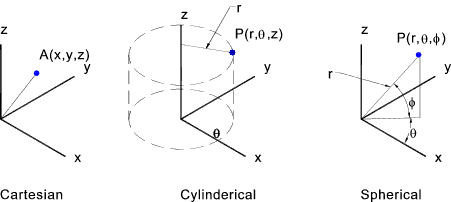Vectors

A vector has magnitude and direction.  A free vector is not defined as regards position.   A localised vector is defined relative to the co-ordinate origin.

Vectors can be manipulated graphically or using algebraic methods.   Generally for 2 dimensional work graphical solutions are more convenient.   For 3 dimensional work it is more convenient to use algebra.

Graphical Methods

Typical methods of adding vectors are shown below..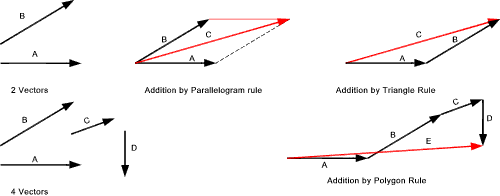Two or more vectors are added to form a RESULTANT.   A single vector can be replaced by separate vectors called COMPONENTS by RESOLUTION of the vector.

The figure above shows how vectors can be obtained, using different methods, to obtain resultant vectors.  It is clearly possible to derive component vectors from a single vector..

Algebraic Methods

It is convenient to represent a vector as a single bold letter e.g.  A.    It is also convenient to separate the magnitude of the vector from its direction.    This is done by use of a unit vector e.g. e which has unit magnitude and has direction.  A is then written as Ae.  The modulus A written as | A | is the size of the vector and is positive.

In the cartesian co-ordinates the unit vectors in the x,y & z directions are identified as i, j & k.    Therefore a vector A can be broken down into its components...

A = A x i + A y j + A z k<

The modulus | A | 2 = A x2 + A y2 + A z2

Adding two vectors A + B is conveniently done by adding the components in the x, y, & z directions.

A + B = (A x + B x) i + (A y+ B y) j + (A z + B z ) k.

It is clear that for free vectors A + B = B + A

Multiplying a vector by a scalar does not alter it direction.

x .A = x. A x i + x. A y j + x. A z k

The scalar product of two vectors A and B is formally defined as |A| .|B| Cos θ where θ is the smallest angle between the vectors..
....An example of a scalar product is when an angled force moves a load. The work don is the scalar product of the vector of the force and the vector of the distance moved by the load

The cross product of two vectors A and B is formally defined as |A| x |B| Sin θ where θ is the smallest angle between the vectors...  The resultant vector is in a direction orthogonal to plane of containing the two manipulated vectors as defined by the right hand rule.
.... An example of a cross product is when a couple results from applying a force at a distance from a point. The resulting torque is identified vectorially as the cross product of the force and distance vectors.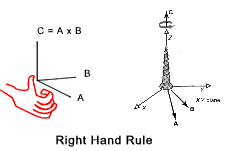The right-hand rule states that the orientation of the vectors' cross product is determined by placing A and B tail-to-tail, flattening the right hand, extending it in the direction of A, and then curling the fingers in the direction that the angle B makes with A.    The thumb then points in the direction of A x B.

To consider a cross product in cartesian co-ordinate form

A x B = (Ax i + Ay j + Az k ) x (Bx i + By j + Bz k )

It can be proved that

i x j = k = - j x i
j x k = i = - k x j
k x i = j = - i x k
i x i = j x j = k x k = 0

This reduces the cross product to

A x B = (A y . B z  —  Az . B y).i   +    (A z . B x   —  A x . B z).j   +    (A x . B y   —  A y . B x).k

This is most compactly expressed as a determinant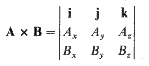General Notes on Vector Operations

Commutative Operations ..... A + B + C = B + C + A = C + B + A
Associative Operations.... (A + B) + C = A + (B + C)
Distributive Operations.... a.(A + B + C) = a.A + a.B + a.C

Triple Scalar Products.... (AxB).C = C.(AxB).. Note . Brackets are really not necessary..

Triple Vector Products.... (AxB)xC = -Cx(AxB)= Cx(BxA)...Note .Brackets and essential

The following relationships are provided

AxB)xC = C.AB - C.BA
Ax(BxC) = A.CB - A.BC... Note:..A.CBis interpreted as a scalar dot product A.C multiplied by a vector B

Differentiation of Vectors

In general the differentiation of vectors follows the same rule as the differentiation of scalar quantities.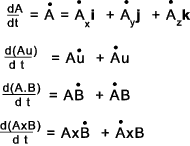Links to Vectors & Forces Vector components and addition..NASA..Short review Vectors - Wolfram mathwold..Definitive detailed information

Mechanics Index